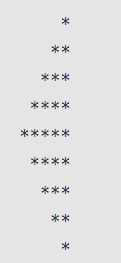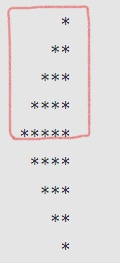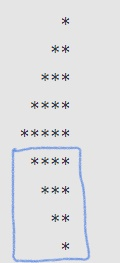Related Tags

communitycreator
javascript

# How to create a left pascal pattern in JavaScript

Ayush Trivedi

### Overview

In this shot, we’ll learn how to create a Left Pascal star pattern. This pattern has a medium level of complexity.

The image shown below is the Left Pascal star pattern, which we will create using JavaScript.### Code

let n = 5;

for (let i = 1; i <= n; i++) {
for (let j = 0; j < n - i; j++) {
process.stdout.write(" ");
}
for (let k = 0; k < i; k++) {
process.stdout.write("*");
}
console.log();
}
for (let i = 1; i <= n - 1; i++) {
for (let j = 0; j < i; j++) {
process.stdout.write(" ");
}
for (let k = 0; k < n - i; k++) {
process.stdout.write("*");
}
console.log();
}

### Explanation

1. To draw the upper part of left pascal triangle marked in red, follow the steps below.• Line 1: We initialize the number for the triangle’s height or the number of rows.

• Line 3: This for loop will iterate for n times that is 5. So the upper part will have 5 rows.

• Line 4: This first nested for loop will print spaces before * for n-i times for the current row(i) of triangle. For example, for i=2 spaces for 2nd row will be 3 and for i=3 that is for 3rd row spaces before star will be 2 and so on.

• Line 7: This second nested for loop will print the * for i times after the spaces, where i is the outer loop iteration which is current row also so if 1st row number of star is 1, for i=2 numberof start is 2 and so on.

• Line 10: We will change the row using console.log(), this will change the current line in the console.

1. To draw the lower part of left pascal triangle, marked in blue follow the steps below.

We have already initialized the n in the upper part. This part will have one less row than the upper part.• Line 12: This for loop will print the lower part of the pattern. It will iterate for n-1 times, that is, 5-1 = 4 times.

• Line 13: This nested for loop is used to print spaces before the stars in a row. The number spaces will be equals to the i(the current iteration or the row for this lower part). So if i=1 that is for the first row of this lower part the spaces will be 1, for i=2 that is 2nd row and spaces will be 2 and so on.

• Line 16: In this nested for loop, it will print the * after the spaces which we print in the above for loop, It will print * n-i times(where n=5, and i is current iteration or the current row). so if i=1 number of * will be 5-1(n-i) = 4, for i=2 number of * will be 5-2(n-i) = 3 and so on.

• Line 19: We will change the row using console.log(), this will change the current line in the console.

### Output

We have created a Left Pascal star pattern in JavaScript.

RELATED TAGS

communitycreator
javascript

CONTRIBUTOR

Ayush Trivedi
RELATED COURSES

View all Courses

Keep Exploring

Learn in-demand tech skills in half the time